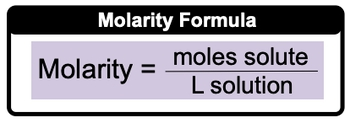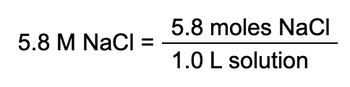## GOB Chemistry

Learn the toughest concepts covered in your GOB - General, Organic, and Biological Chemistry class with step-by-step video tutorials and practice problems.

Solutions

# Molarity

Ever wonder how a mass amount such as moles can be converted into the volume amount of liters? Well, molarity serves as the bridge between moles and liters.

Molarity
1
concept

## Molarity53s
Play a video:
in these series of videos, we're gonna take a look at the concept of polarity, not morality. Itself is represented by the variable M and in class you'll hear more clarity and concentration, sometimes being used interchangeably. Remember, concentration represents the amount of given salute within a certain amount of solution. Now, mole Arat E is more specific. Molar ity represents the number of moles of solute per leader of solution. And with this we have our polarity formula similarity, which is capital m equals moles off salute over leaders off solution. Now that we have, um, or fleshed out idea of what concentration is due morality, let's take a look at some problems or ask the conflict polarity or to look at the components that help to make more clarity.2
example

## Molarity Example 11m
Play a video:
here, it says, to calculate the molar ity of a solution prepared by dissolving 23. g of sodium hydroxide in enough water to make 2. liters of solution. So here we're looking for more clarity. So mole arat E. Here equals are moles of solute, which would be the H. And it is. It's in a solution off 2.0 leaders, so we need leaders off solution. All right, we already have the 2.50 liters of solution, so we just need to convert the grams of sodium hydroxide into moles. So one mole of sodium hydroxide goes on the bottom, and then grams of sodium hydroxide goal. One more goes on the top and grams of sodium hydroxide go on the bottom. Sodium hydroxide is composed off one sodium, one oxygen and one hydrogen. Multiply them by their atomic masses from the periodic table, okay and then add up the totals. This will give us the Moller mask of sodium hydroxide, so we add that all up, it's 39.998 g, so that's going to go here. So here grams of sodium hydroxide cancel out and Now I'm gonna have moles of sodium hydroxide. So that comes out 2.59 to 5 moles. Take that and plug it in and we'll have the mole polarity of our solution. So this comes out 2. Moeller for solution.
3
concept

## Molarity1m
Play a video:
Now that we know what polarity is, we can use it to help us calculate unknowns. We're gonna say problems with the polarity value present can use a given amount and conversion factors to isolate and end amount. Now, if you haven't watched our videos on dimensional analysis and conversion factors, I highly suggest you go back and take a look because it's just a continuation of this. We're now we're incorporating, incorporating more clarity into our calculations. Now the polarity value itself can represent one of those conversion factors. Because remember, conversion factors possess two different units connected together. Now, for example, we say 5.8 Moeller sodium chloride 5.8 Moeller represents 5.8 moles of N a. C L. Within one leader of solution. And because we're using two different units connected together, this is a conversion factor, so we can write it as 58 moles of N a. C. L. Over one leader of solution. So this is what 5.8 Moeller really represents. So keep that in mind when we start doing calculations that incorporate malaria4
example

## Molarity Example 23m
Play a video:
5
Problem

What volume in (µL) of 0.125 M HBr contains 0.170 moles HBr?

6
Problem

Hypernatremia is a medical condition where a patient has high levels of sodium in their blood, and is the result of the body containing too little water. A patient has a measured sodium level of 165 mM. If 30.0 mL of their blood were drawn, what mass (in ng) of sodium would be present?

7
Problem

2.64 grams of an unknown compound was dissolved in water to yield 150 mL of solution. The concentration of the solution was 0.075 M. What was the molecular weight of the substance?

8
Problem

A solution with a final volume of 750.0 mL was prepared by dissolving 30.00 mL of benzene (C6H6, density = 0.8787 g/mL) in dichloromethane. Calculate the molarity of benzene in the solution.